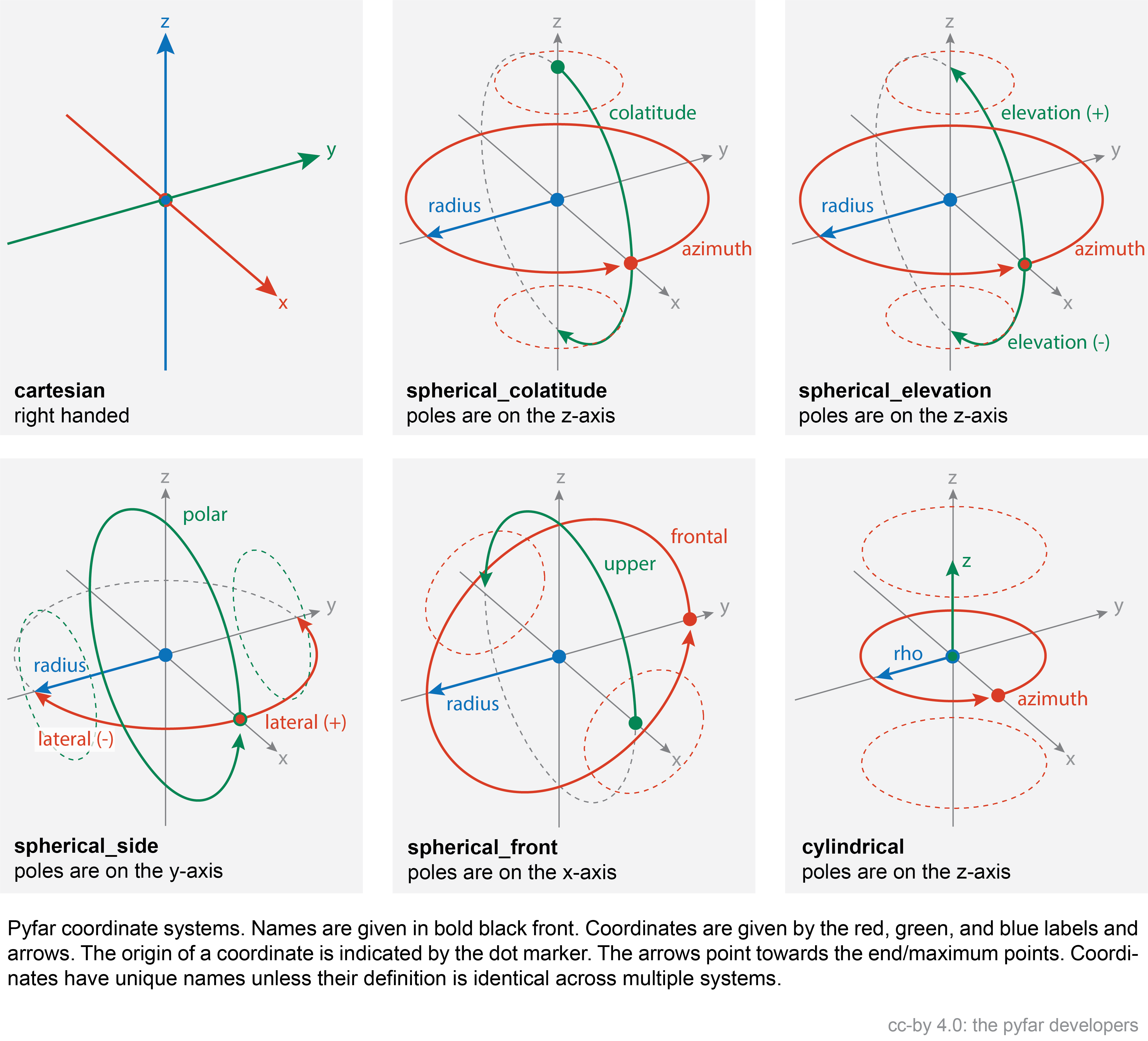# Coordinates¶

The following introduces the concept of the coordinates class and the coordinate systems that are available in pyfar.

## Coordinates Class¶

Different coordinate systems are frequently used in acoustics research and handling sampling points and different systems can be cumbersome. The Coordinates class was designed with this in mind. It can convert between coordinate systems and rotate, query and plot coordinates points. Functions for converting coordinates not stored in a Coordinates object are available for convenience. However, it is strongly recommended to use the Coordinates class for all conversions.

## Coordinate Systems¶

Coordinate systems are defined by their domain (e.g. 'spherical'), convention (e.g. 'top_elev'), and unit (e.g. 'deg'). The available coordinate systems are shown in the image belowThe unit for length is always meter, while the unit for angles is radians. For more details see the table below.

Coordinate

Descriptions

x, y, z

x, y, z coordinate of a right handed Cartesian coordinate system in meter ($$-\infty$$ < x,y,z < $$\infty$$).

azimuth

Counter clock-wise angle in the x-y plane of the right handed Cartesian coordinate system in radians. $$0$$ radians are defined in positive x-direction, $$\pi/2$$ radians in positive y-direction and so on ($$-\infty$$ < azimuth < $$\infty$$, $$2\pi$$-cyclic).

elevation

Angle in the x-z plane of the right handed Cartesian coordinate system in radians. $$0$$ radians elevation are defined in positive z-direction, $$\pi/2$$ radians in positive x-direction, and $$\pi$$ in negative z-direction ($$\pi/2$$ < elevation < $$\pi/2$$). The elevation is a variation of the elevation angle.

colatitude

Angle in the x-z plane of the right handed Cartesian coordinate system in radians. $$0$$ radians colatitude are defined in positive x-direction, $$\pi/2$$ radians in positive z-direction, and $$-\pi/2$$ in negative z-direction ($$0$$ < colatitude < $$\pi$$). The colatitude is a variation of the colatitude.

lateral

Counter clock-wise angle in the x-y plane of the right handed Cartesian coordinate system in radians. $$0$$ radians are defined in positive x-direction, $$\pi/2$$ radians in positive y-direction and $$-\pi/2$$ in negative y-direction ($$-\pi/2$$ < lateral < $$\pi/2$$).

polar

Angle in the x-z plane of the right handed Cartesian coordinate system in radians. $$0$$ radians elevation are defined in positive x-direction, $$\pi/2$$ radians in positive z-direction, $$\pi$$ in negative x-direction and so on ($$-\infty$$ < polar < $$\infty$$, $$2\pi$$-cyclic).

frontal

Angle in the y-z plane of the right handed Cartesian coordinate system in radians. $$0$$ radians elevation are defined in positive y-direction, $$\pi/2$$ radians in positive z-direction, $$\pi$$ in negative y-direction and so on ($$-\infty$$ < frontal < $$\infty$$, $$2\pi$$-cyclic).

upper

Angle in the x-z plane of the right handed Cartesian coordinate system in radians. $$0$$ radians elevation are defined in positive x-direction, $$\pi/2$$ radians in positive z-direction, and $$\pi$$ in negative x-direction ($$0$$ < upper < $$\pi$$).

radius

Distance to the origin of the right handed Cartesian coordinate system in meters ($$0$$ < radius < $$\infty$$).

rho

Distance perpendicular to the the z-axis of the right handed Cartesian coordinate system ($$0$$ < rho < $$\infty$$).

## Samplings¶

A plethora of sampling schemes to generate coordinate objects is contained in samplings.